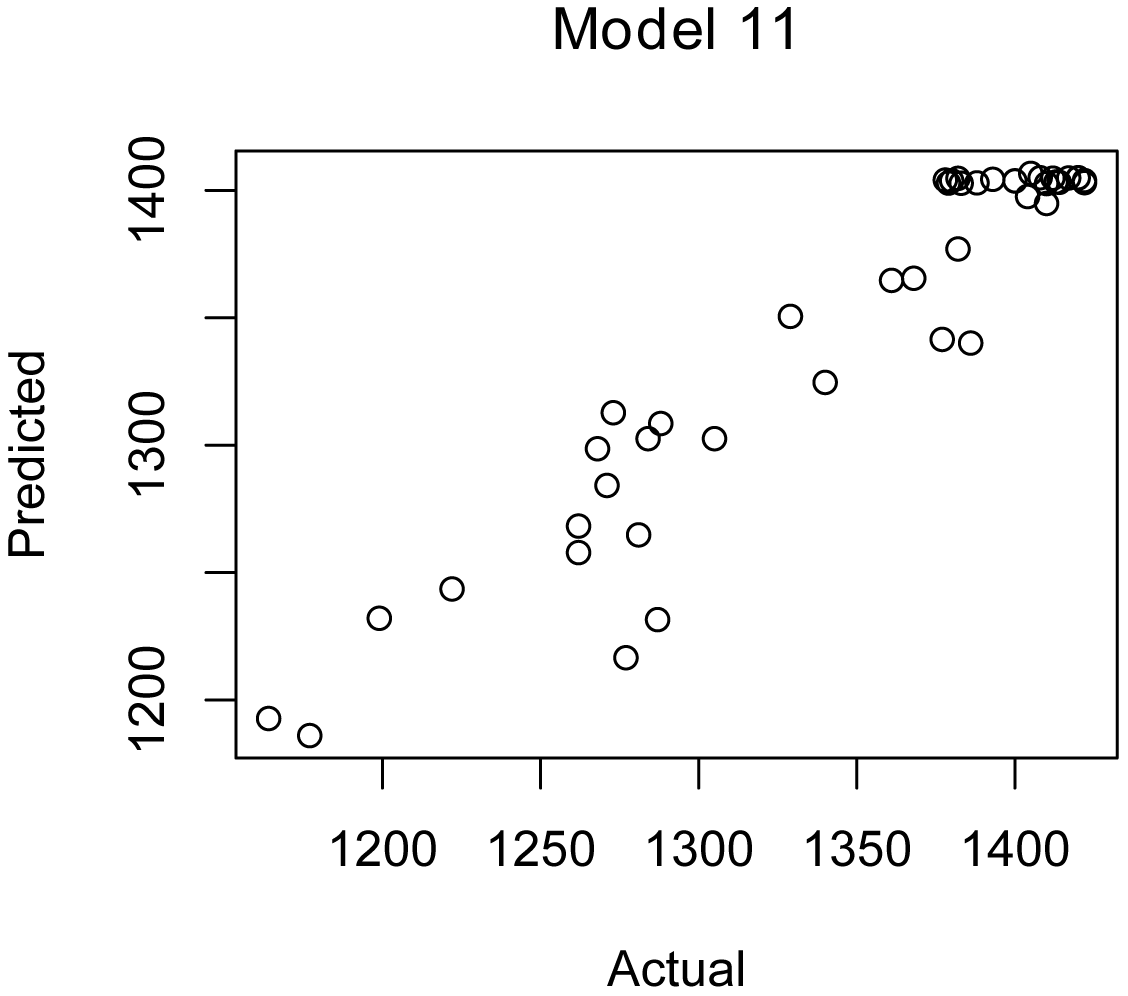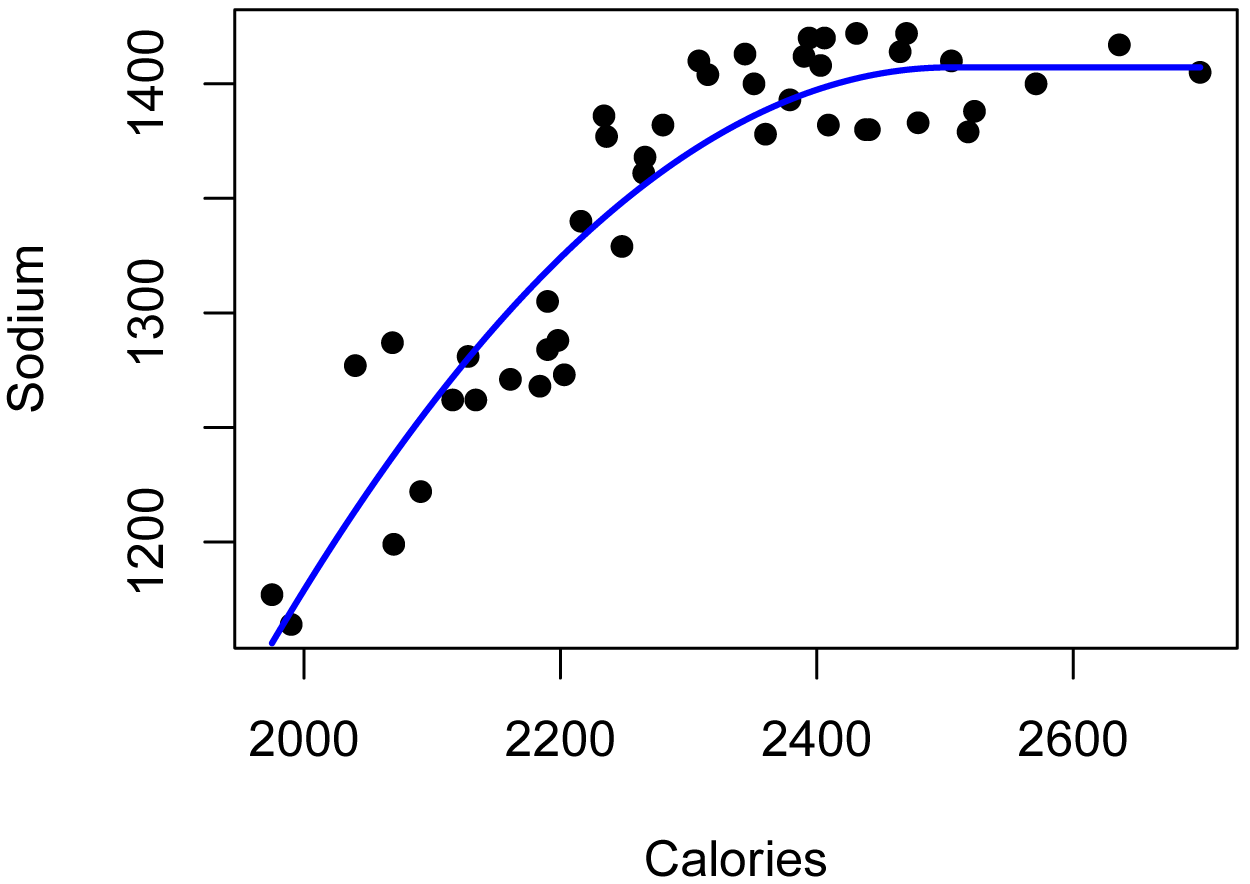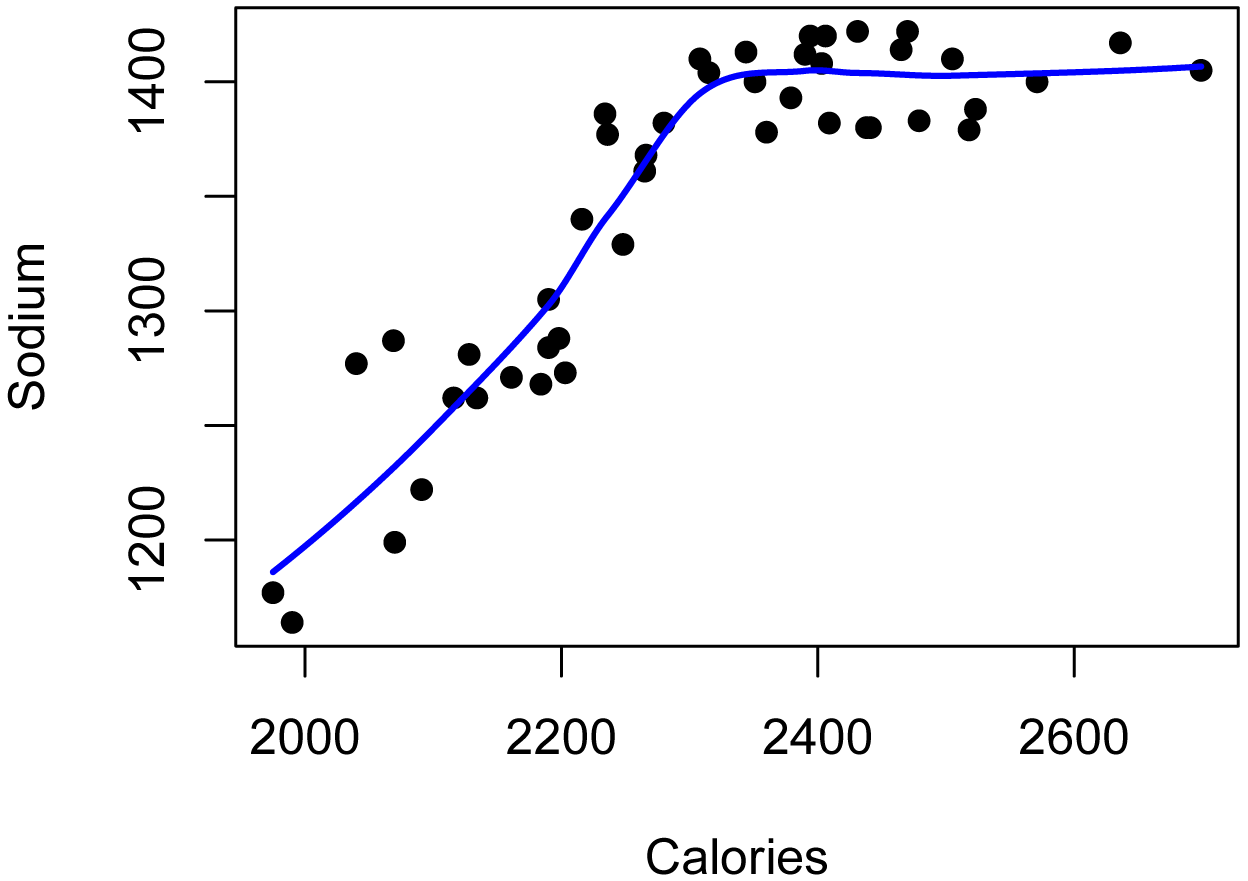## Summary and Analysis of Extension Program Evaluation in R

Salvatore S. Mangiafico

# Accuracy and Errors for Models

Measures of accuracy and error can be used to determine how well a given model fits the data.   They are distinct from the R-squared and pseudo R-squared measures discussed in the last chapter.

These statistics are useful to compare a wide variety of models where the dependent variable is continuous.  In general, one would want to compare only among models with the same data for the dependent variable.

 Measure Units Interpretation Min.max.accuracy Unitless 1 is perfect fit Mean absolute error    (MAE) Same as variable 0 is perfect fit Mean absolute percent error    (MAPE) Unitless 0 is perfect fit Mean square error    (MSE) Square of variable units 0 is perfect fit Root mean square error    (RMSE) Same as variable 0 is perfect fit Normalized root mean square error    (NRMSE) Unitless 0 is perfect fit NRMSE Accuracy Unitless 1 is perfect fit Efron’s R-squared Unitless 1 is perfect fit

### Packages used in this chapter

The packages used in this chapter include:

•  rcompanion

•  betareg

•  nlme

•  lme4

•  quantreg

•  mgcv

•  MASS

•  robust

The following commands will install these packages if they are not already installed:

if(!require(rcompanion)){install.packages("rcompanion")}
if(!require(betareg)){install.packages("betareg")}
if(!require(nlme)){install.packages("nlme")}
if(!require(lme4)){install.packages("lme4")}
if(!require(quantreg)){install.packages("quantreg")}
if(!require(mgcv)){install.packages("mgcv")}
if(!require(MASS)){install.packages("MASS")}
if(!require(robust)){install.packages("robust")}

### Examples of accuracy and error

The following example fits various models to the BrendonSmall data set in the rcompanion package, and then uses the accuracy function to produce accuracy and error statistics for these models.

Note that model.5 has a different dependent variable than the others.

### Prepare data set

library(rcompanion)

data(BrendonSmall)

BrendonSmall\$Calories = as.numeric(BrendonSmall\$Calories)
BrendonSmall\$Sodium = as.numeric(BrendonSmall\$Sodium)
BrendonSmall\$Calories2 = BrendonSmall\$Calories ^ 2
BrendonSmall\$Sodium.ratio = BrendonSmall\$Sodium / 1500

Instructor Grade Weight Calories Sodium Score Calories2 Sodium.ratio
1 Brendon Small     6     43     2069   1287    77   4280761    0.8580000
2 Brendon Small     6     41     1990   1164    76   3960100    0.7760000
3 Brendon Small     6     40     1975   1177    76   3900625    0.7846667
4 Brendon Small     6     44     2116   1262    84   4477456    0.8413333
5 Brendon Small     6     45     2161   1271    86   4669921    0.8473333
6 Brendon Small     6     44     2091   1222    87   4372281    0.8146667

### Fit various models

model.1 = lm(Sodium ~ Calories, data = BrendonSmall)

model.2 = lm(Sodium ~ Calories + Calories2, data = BrendonSmall)

model.3 = glm(Sodium ~ Calories, data = BrendonSmall, family="Gamma")

quadplat = function(x, a, b, clx) {
ifelse(x  < clx, a + b * x   + (-0.5*b/clx) * x   * x,
a + b * clx + (-0.5*b/clx) * clx * clx)}
model.4 = nls(Sodium ~ quadplat(Calories, a, b, clx),
data = BrendonSmall,
start = list(a   = 519,
b   = 0.359,
clx = 2300))

library(betareg)
model.5 = betareg(Sodium.ratio ~ Calories, data = BrendonSmall)

library(nlme)
model.6 = gls(Sodium ~ Calories, data = BrendonSmall, method="REML")

model.7 = lme(Sodium ~ Calories,
random = ~1|Instructor,
data = BrendonSmall,
method="REML")

library(lme4)
model.8 = lmer(Sodium ~ Calories + (1|Instructor),
data=BrendonSmall,
REML=TRUE)

library(lme4)
model.9 = suppressWarnings(glmer(Sodium ~ Calories + (1|Instructor),
data=BrendonSmall,
family = gaussian))

library(quantreg)
model.10 = rq(Sodium ~ Calories,
data = BrendonSmall,
tau = 0.5)

model.11 = loess(Sodium ~ Calories,
data = BrendonSmall,
span = 0.75,
degree=2,
family="gaussian")

library(mgcv)
model.12 = gam(Sodium ~ s(Calories),
data = BrendonSmall,
family=gaussian())

library(MASS)
data = BrendonSmall)

library(robust)
data = BrendonSmall,
family = "poisson")

### Produce accuracy and error statisitcs

library(rcompanion)

accuracy(list(model.1, model.2, model.3, model.4, model.5, model.6,
model.7, model.8, model.9, model.10, model.11, model.12,
model.13, model.14),
plotit=TRUE, digits=3)

\$Fit.criteria
Min.max.accuracy      MAE   MAPE      MSE     RMSE NRMSE.mean NRMSE.median NRMSE.mean.accuracy NRMSE.median.accuracy Efron.r.squared
1             0.976  32.7000 0.0244 1.44e+03  38.0000     0.0282       0.0275               0.972                 0.972           0.721
2             0.983  22.6000 0.0170 7.13e+02  26.7000     0.0198       0.0194               0.980                 0.981           0.862
3             0.975  34.4000 0.0257 1.63e+03  40.4000     0.0300       0.0293               0.970                 0.971           0.684
4             0.984  22.0000 0.0166 7.00e+02  26.5000     0.0196       0.0192               0.980                 0.981           0.865
5             0.980   0.0179 0.0201 4.09e-04   0.0202     0.0225       0.0220               0.977                 0.978           0.822
6             0.976  32.7000 0.0244 1.44e+03  38.0000     0.0282       0.0275               0.972                 0.972           0.721
7             0.979  28.5000 0.0213 1.15e+03  33.9000     0.0252       0.0246               0.975                 0.975           0.778
8             0.979  28.5000 0.0213 1.15e+03  33.9000     0.0252       0.0246               0.975                 0.975           0.778
9             0.979  28.5000 0.0213 1.15e+03  33.9000     0.0252       0.0246               0.975                 0.975           0.778
10            0.976  32.6000 0.0243 1.50e+03  38.7000     0.0287       0.0280               0.971                 0.972           0.710
11            0.987  17.9000 0.0135 5.03e+02  22.4000     0.0167       0.0163               0.983                 0.984           0.903
12            0.987  17.1000 0.0129 4.53e+02  21.3000     0.0158       0.0154               0.984                 0.985           0.913
13            0.979  27.7000 0.0213 1.61e+03  40.1000     0.0298       0.0290               0.970                 0.971           0.690
14            0.743 343.0000 0.2570 1.87e+05 433.0000     0.3210       0.3140               0.679                 0.686         -35.200

Note in the previous results that model.14, the glmRob model, did not fit the data well.  In this case, fitting a Poisson regression model is probably not appropriate for the data here, but is included since this type of model is accepted by the accuracy function.

It is useful to examine plots of the predicted values vs. the actual values to see how well the model reflects the actual values, and to see if patterns in the plots suggest another model may be better.The accuracy measures produced here are different in magnitude than their corresponding R-squared or pseudo R-squared measures.  The following plots and captions illustrate this point.

plotPredy(data  = BrendonSmall,
x     = Calories,
y     = Sodium,
model = model.1,
xlab  = "Calories",
ylab  = "Sodium")

summary(model.1)Plot of model.1, a linear OLS model.  R-squared = 0.721.  Accuracy with RMSE / median = 0.972.

plotPredy(data  = BrendonSmall,
x     = Calories,
y     = Sodium,
model = model.4,
xlab  = "Calories",
ylab  = "Sodium")

nullfunct = function(x, m){m}

m.ini    = 1300

null = nls(Sodium ~ nullfunct(Calories, m),
data = BrendonSmall,
start = list(m = m.ini),
trace = FALSE,
nls.control(maxiter = 1000))

nagelkerke(model.4, null)Plot of model.4, a nonlinear regression model.  Nagelkerke pseudo R-squared = 0.865.  Accuracy with RMSE / median = 0.978.

plotPredy(data  = BrendonSmall,
x     = Calories,
y     = Sodium,
model = model.11,
xlab  = "Calories",
ylab  = "Sodium")Plot of model.11, a loess model.  Accuracy with RMSE / median = 0.984.